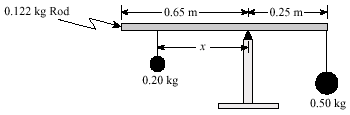# Static equilibrium mass problem

#### brentwoodbc

1. The problem statement, all variables and given/known data
A uniform 0.122 kg rod of 0.90 m length is used to suspend two masses as shown.
At what distance x should the 0.20 kg mass be placed to achieve static equilibrium?2. The attempt at a solution

F1=1.96N
F2=4.9N

torque = 4.9 x 0.25 = 1.225 Nm.cw

1.23 = 1.96r

r=0.63m

answer is actually 0.5

I think its because of the force of 1.96 (0.20kg) being to the right of the end of the rod (which has a mass)

Last edited:

#### tiny-tim

Homework Helper
Welcome to PF!

Hi brentwoodbc! Welcome to PF!F1=1.96N
F2=4.9N
What about F3 (for the rod)?

(And please don't multiply everything by 9.8 …

just call it g … all the g's wil cancel in the end, anyway)

#### brentwoodbc

Re: Welcome to PF!

Hi brentwoodbc! Welcome to PF!What about F3 (for the rod)?

(And please don't multiply everything by 9.8 …

just call it g … all the g's wil cancel in the end, anyway)

thanks, ya Im trying to figure out how to factor in the force of the rod but we were not given any example where the rod had a mass.

#### tiny-tim

Homework Helper
… we were not given any example where the rod had a mass.
ok … the rod has a weight …

where does that weight act?#### brentwoodbc

Re: Equalibrium

ok … the rod has a weight …

where does that weight act?on the centre of rotation. and the sum of all forces = zero. What direction is the 3rd force though? against the other two?

#### tiny-tim

Homework Helper
on the centre of rotation. and the sum of all forces = zero. What direction is the 3rd force though? against the other two?
(sorry, i'm not following you)

the weight of a rod (or indeed anything else) acts through its centre of mass

#### brentwoodbc

Re: Equalibrium

so whats the equation going to look like?

F1+F2+F3=0?

Im lost on this question.

#### tiny-tim

Homework Helper
No, torque1 + torque2 + torque3 = 0#### brentwoodbc

Re: Equalibrium

ok, but I keep getting -0.35

.5gr+.2gr+.122gr=0

.2gr=-(.122g(.45 -.25)+.5(.25))

cancel g

divide .2

r = 0.75?

#### tiny-tim

Homework Helper
ok, but I keep getting -0.35

.5gr+.2gr+.122gr=0

.2gr=-(.122g(.45 -.25)+.5(.25))

cancel g

divide .2

r = 0.75?
curiously, that seems to be the right answer …

but what was the (.45 -.25) supposed to be?#### brentwoodbc

Re: Equalibrium

curiously, that seems to be the right answer …

but what was the (.45 -.25) supposed to be?half of distance of beam is 0.45metres

so minus the 0.25metres is the distance of the centre of mass from the centre of rotation so (r for F3)

#### brentwoodbc

Re: Equalibrium

I noticed that the correct answer "0.5"is my answer "0.75" minus the 0.25 to the right of the centre of rotation... hmmm.

#### tiny-tim

Homework Helper
ah … got it!

that's fine then#### brentwoodbc

Re: Equalibrium

ah … got it!

that's fine thenIm not 100% but I think that since the force with mass.5 is clockwise "in direction" it is negative. the other two are in a counter clockwise direction. So I made the 0.25metres negative and I got 0.5.

Seems to be fine. Thank you very much.#### tiny-tim

Homework Helper
I noticed that the correct answer "0.5"is my answer "0.75" minus the 0.25 to the right of the centre of rotation... hmmm.
oh, I missed the minus in your
.2gr=-(.122g(.45 -.25)+.5(.25))
that does make it .5#### brentwoodbc

Re: Equalibrium

oh, I missed the minus in your

that does make it .5pfft. I'm so smart lolthanks again though. Being spring break I cant get help and I have tests a couple days after I go back. And there are more question s in my homework similar to this so I should be cool now.

### The Physics Forums Way

We Value Quality
• Topics based on mainstream science
• Proper English grammar and spelling
We Value Civility
• Positive and compassionate attitudes
• Patience while debating
We Value Productivity
• Disciplined to remain on-topic
• Recognition of own weaknesses
• Solo and co-op problem solving